# Quiz 14: Sound

Physics & Astronomy

The buzzing sounds of the flying insects are due to the rapid motion of the wings of the insect. For example, honey bees flap their wings 11,400 times per minute, resulting in a large buzzing sound. If the frequency of flap of wings is in the audible range that is from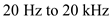,, then those sounds will be audible to us. Otherwise, they are not audible even if the frequency of flapping is very high. Therefore, it is confirmed that flapping of wings of some insects produce sounds will be audible to us.

Speed of the sound wave depends upon the temperature of the particular medium. By increasing in the temperature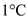, the speed of sound increases by about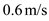and it can be expressed mathematically as,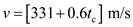(a) Speed of sound with rise of temperature is,Substitute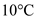for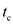in the above equation.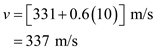Therefore, the velocity of sound atis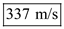. (b) Speed of sound with rise of temperature is,Substitute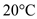forin the above equation.Therefore, the velocity of sound atis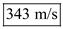.

Audible sound frequency ranges from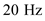to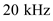. Sound with frequency greater thanis called ultrasonic. Supersonic is a wave which has a speed greater than that of the sound. Therefore, the options (a), (c), and (d) are incorrect. Infrasonic sound frequency is below.therefore, sound wave with frequency of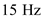is in the region of infrasonic sound. Therefore, the correct answer is option (b).

There is no answer for this question